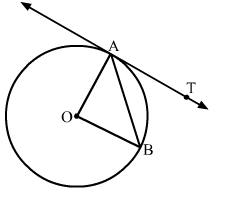# In the given figure, O is the centre of the circle AB is a chord and AT is the tangent at A.`
Question:

In the given figure, O is the centre of the circle AB is a chord and AT is the tangent at A. If ∠AOB = 100 then ∠BAT is equal to

(a) 40
(b) 50
(c) 90
(d) 100Solution:

Given: AO and BO are the radius of the circle
Since, AO = BO
∴ △AOB is an isosceles triangle.
Now, in △AOB
∠AOB + ∠OBA + ∠OAB = 180        (Angle sum property of triangle)
⇒ 100  + ∠OAB + ∠OAB = 180      (∠OBA = ∠OAB)
⇒ 2∠OAB = 80
⇒ ∠OAB = 40
We know that the radius and tangent are perperpendular at their point of contact
∵∠OAT = 90
⇒ ∠OAB  + ∠BAT = 90
⇒ ∠BAT = 90 − 40 = 50
Hence, the correct answer is option (b).